Next: The Raw Asymmetry For 2-Counter Geometry Up: Measuring the penetration depth with TF-SR Previous: The Field Distribution

# The Role of the Positive Muon

The elegance of the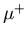SR technique is the employment of a positive muonto microscopically probe the local magnetic field distribution of Eq. (3.1) in the bulk of the superconducting sample. Thus, measurements of the penetration depth usingSR are less sensitive to the sample's surface than other conventional techniques which measure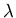. A positive muon has the following properties [77,79]:

Rest mass: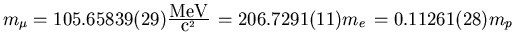Charge: +e
Spin: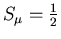Magnetic Moment: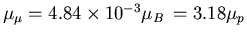Average Lifetime: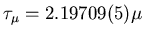s
Muon Gyromagnetic Ratio: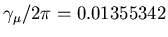(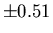ppm)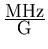Muons are generated via the weak decay of pions: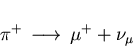(12)

A low energy-beam can be created by making use of the fact that some of the pions which are produced in collisions of high energy protons with a fixed carbon or beryllium production target stop inside that target. These pions rapidly decay (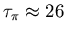ns) according to Eq. (3.12). Muons produced in this manner are often referred to as surface muons to distinguish them from higher momentum muons which are obtained from pions which decay while in flight. Aemerging from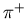-decay has the direction of its spin antiparallel to its momentum vector. This result follows from conservation of linear and angular momentum, and the fact that the neutrino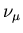is a left-handed particle (i.e. the neutrino helicity = -1). A beamline composed of magnetic dipole and quadrupole magnets is used to collect muons emitted in a given direction. Consequently, these muons are nearly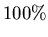spin polarized, with their spin pointing backwards relative to their momentum.

In the TF-SR arrangement (see Fig. 3.10), thespin is rotated perpendicular to the muon momentum using a Wien filter or separator, which consists of mutually perpendicular electric and magnetic fields, both of which are transverse to the beam direction. After passing through a collimator, the spin-polarized positive muons are implanted one by one into the sample, which is situated in an external field H. The applied field is transverse to the initial muon spin polarization, and hence parallel to the muon momentum. The muons entering the sample rapidly thermalize (stop) within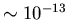s. Their low momentum implies a short range in the sample: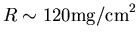, which corresponds to a thickness of approximately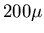m in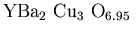.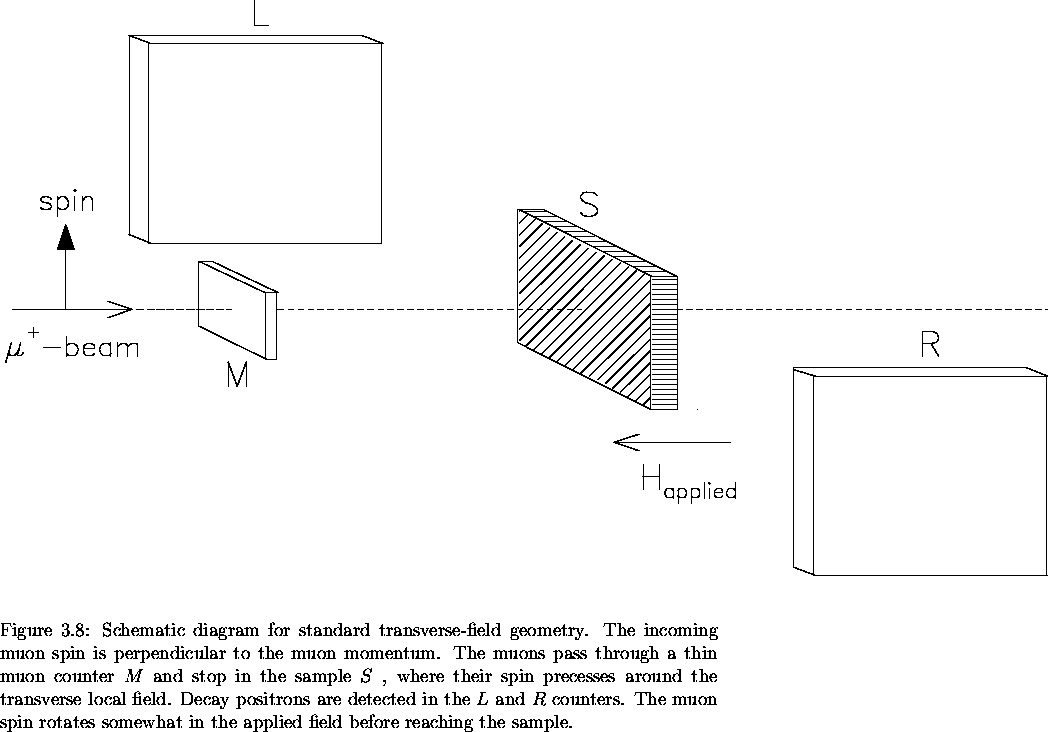Inside the sample, the muon's magnetic moment comes under the influence of the local magnetic field. For, the local magnetic field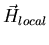is the vector sum of the externally applied field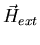, the magnetic field produced by the circulating supercurrents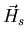, and the field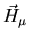resulting from local magnetic moments: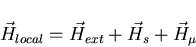(13)

The muons precess about the local fieldwith a Larmor frequency: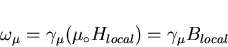(14)

where Blocal is the local magnetic induction and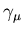is the muon gyromagnetic ratio. If the muons occupy identical sites in the sample, they will be subjected to the same local magnetic field. Therefore they will precess in phase with one another so that the magnitude of their initial polarization is retained. If, however, the muons experience different magnetic fields in the sample, they will not precess at the same Larmor frequency. The resulting dephasing of their spins manifests itself in a corresponding loss of polarization. After a mean lifetime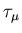, thedecays into a positron and two neutrinos: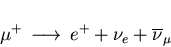(15)

The distribution of decay positrons is asymmetric with respect to the spin polarization vector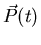of the muon, such that the highest probability of emission is along the direction of the muon spin. Consequently, the time evolution of the muon spin polarizationcan be monitored, since thereveals its spin direction at the time of decay.

A thin-detector placed in front of the sample registers the arrival of a muon in the sample and starts a clock. A pair of positron detectors (one on either side of the sample as in Fig. 3.10) can be used to detect the positrons emerging from the-decay. Once a decay positron is registered, the clock is stopped. For a single counter the number of positrons recorded per time bin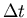is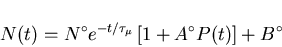(16)

where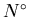is a normalization constant,is the muon lifetime,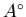is the maximum precession amplitude (or maximum experimental decay asymmetry), P(t) is the time evolution of the muon spin polarization in the direction of the positron counter and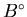is a time-independent background from unwanted events.Next: The Raw Asymmetry For 2-Counter Geometry Up: Measuring the penetration depth with TF-SR Previous: The Field Distribution
Jess H. Brewer
2001-09-28